@sticky-now @sticky-add: @:libyli @:title-slide /no-status # // comment- {{ nd.vars.author }} {.no} - {.no} - Talk at {{ nd.vars.venue }}, {{ nd.vars.date }} {.no} @for-copy @:#overview overview no-libyli ## Overview - Introduction - Anomaly and fraud detection - Imbalanced classification problems - The Problem (and performance measures) - Reweight, resampling, etc - Learning maximum excluding ellipsoids - Correcting k-NN: *$\gamma$-NN@:accro* - Focusing on the F-Measure optimization - Probabilistic models for unsupervised anomaly detection - Discussion @eval-header: return highlightLi(1) # @copy: overview @:.denser /no-status ## Laboratoire Hubert Curien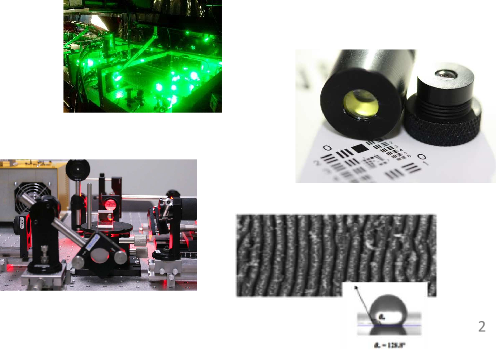- Optic-Photonic & Microwaves: - Micro & nano structuration - Laser Processes - Materials & Surfaces - Functionalization of surfaces - Materials for harsh environments - Informatics, Telecom & Image: - Images Analysis - Data intelligence - Secured embedded Systems @:.dense ## “Data Intelligence” Team:
**Machine Learning and Complex Data Analysis**{.dense}The team is specialized in statistical machine learning and data analysis and addresses mainly the following areas: - **Representation Learning:** Deep Learning, Embedding for structured data (graphs, texts, images, sequences), incorporation of background knowledge and interpretability. - **Metric Learning:** optimizing ad hoc metrics (distance/similarity) under semantic constraints. - **Transfer Learning and Domain Adaptation:** Adapting and transferring models to new tasks or domains. Metric Learning - **Learning theory:** Developing theoretical guarantees, formal frameworks and interpretation for learned models. (PAC-Bayesian Theory, Optimal Transport, ...) @:.dense ## “Data Intelligence” Team:
**Machine Learning and Complex Data Analysis**{.dense}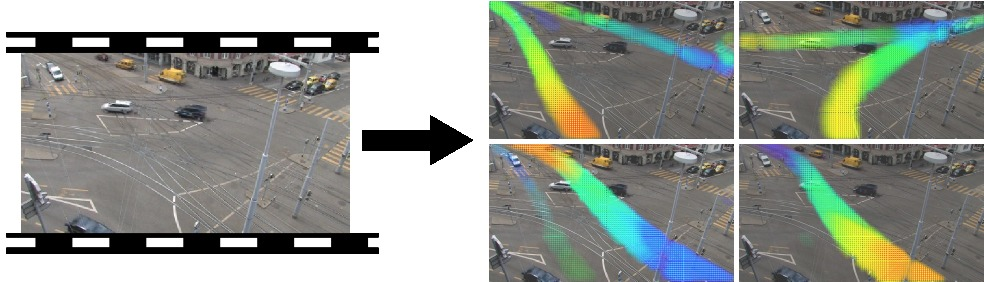- **Data Mining:** Designing large scale methods to extract relevant and meaningful information from structured data, such as graphs or sequences, in the form of frequent or rare (spatio-temporal) patterns. - **Learning/Analyzing from difficult scenarios:** Dealing with highly imbalanced data, few learning samples, incomplete data, privacy and fairness constraints. - **Flagship Applications:** - Anomaly and Fraud Detection - Computer Vision - Medical data Analysis - Textual Data Analysis - Social Network Analy @@@@@@@@@@ INTRO TWO APPROACHES @@@@@@@@@@ @eval-header: return highlightLi(2) # @copy: overview ## Supervised / Unsupervised *Machine Learning*{.dense .step}@anim: #sup | #suppoints | #unsup,#unsuppoints | #separator | #groups | #voronoi @: .dense ## Supervised vs Unsupervised Learning - Supervised - Given example inputs ($X$) and corresponding outputs ($y$) - Learn the fonction “input → output” ($y = f(X)$) - classification (categorical output) - regression (continous output) - methods: - k-NN, SVM, SVR, Random Forests, Least Squares fit, {.no} - Neural Networks, Gaussian Processes, Boosting, ... {.no} - Unsupervised - Given a set of data points ($x$) - Model/structure/understand this dataset - clustering, densitiy estimation - source separation - pattern and sequence mining - rare events / anomaly detection - Methods: - PCA, k-means, OneClass-SVM, Isolation Forests, PGM (GMM, HMM, ...), {.no} - DBSCAN, Autoencoders, GANs, KDE ... {.no} ## Sup. / Unsup. *Anomaly Detection*{.dense .step}@anim: #sup | #suppoints | #unsup,#unsuppoints | #separator | #groups | #supqueries | #unsupqueries # Class Imbalance or Novelty???@@@@@@@@@@ IMBALANCED @@@@@@@@@@ @eval-header: $o.s = 3 @eval-header: return highlightLi($o.s, 1) # @copy: overview ## Imbalanced Problems: Examples // generally, but at the lab - Anomaly detection *// incl images* - unsafe situations in videos // bluecime, first step into it for me - defect detection in images // often as out-of-distribution though - abnormal heart beat detection in ECG - Fraud detection - fraudulent checks - credit card fraud (physical, online) - financial fraud (French DGFIP) // Dir. Géné. des Fin. Pub. - @:displaynone // TODO: add illustr ## Imbalanced Classification Problems // generally, but at the lab- Binary classification - $+$ positive class: minority class, anomaly, rare event, … {.no} - $-$ negative class: majority class, normality, typical event, … {.no} - Confusion matrix (of a model vs a ground truth) - TP: true positive - FP: false positive - TN: true negative - FN: false negative - @anim: #predp | #tpetal - Some measures {.padli .densemath} - Precision: $prec=\frac{TP}{TP+FP}$ - Recall: $rec=\frac{TP}{P} = \frac{TP}{TP+FN}$ - $F_\beta$-measure: $F_\beta = (1+\beta^2)\frac{prec\cdot rec}{\beta^2 \cdot prec + rec}$ \ *(higher is better)*{.dense} ## F-measure vs Accuracy ? - $F_\beta = (1+\beta^2)\frac{prec\cdot rec}{\beta^2 \cdot prec + rec} = \frac{(1+\beta^2)\cdot (P - FN)}{1 + \beta^2 P - FN + FP}$ \
{.no} - $accuracy = \frac{TP + TN}{P+N} = 1 - \frac{FN+FP}{P+N}$
{.no} - Accuracy inadequacy (e.g. $N=10000, P=100$) - lazy "all$-$" classifier ($TP=0, TN=N, FP=0, FN=P$) - $\textstyle \textcolor{orange}{accuracy} = \frac{0 + N}{P + N} = \frac{10000}{10100} \textcolor{orange}{= 99\\%}$ - $\textstyle \textcolor{orange}{F_\beta} = \frac{(1+\beta^2) (P - P)}{1 + \beta^2 P - P + 0} \textcolor{orange}{= 0}$ - $F_\beta$-measure challenges - discrete (like the accuracy) - non-convex (even with continuous surrogates) - **non-separable**, i.e.    $F_\beta \ne \sum_{(x_i, y_i) \in S}...$ // TODO maybe a schema for the trivial case ## Ok, but… I'm doing gradient descent, so…- Gradient:   $0.2$ ⇒ $-7.21$,   $0.5$ ⇒ $-2.89$,   $0.8$ ⇒ $-1.80$,    $1$ ⇒ $-1.44$ - Example, gradient intensity is the same for: - $10$ $+$ wrongly classified with an output proba. of $0.2$ - $40$ $-$ correctly classified with an output proba $0.8$ - i.e., lazily predicting systematically $0.2$ (for $+$)
yields a "stable" solution with $10+$ vs $40-$ ## Ok, but… my deep model does 100%… - ... the 100% accuracy is on the train set - ... I cannot tell you if it will generalize well - Our team is working on these aspects - [APRIORI ANR project](https://project.inria.fr/apriori/) - guarantees for deep representation learning @eval-header: return highlightLi($o.s, 2) # @copy: overview ## Counteracting Imbalance - Undersampling the majority class$-$- Oversampling class$+$- Generating fake$+$- Using a weighted-classifiers learner // For models that learn, can reweigh but not perfect (ideal ratio depends on Bayesian error and régularisation etc) can x valid? @eval-header: return highlightLi($o.s, 3) # @copy: overview @:paperattrib #gammann no-libyli ## Learning maximum excluding ellipsoids from imbalanced data with theoretical guarantees - **Guillaume Metzler**, Xavier Badiche, Brahim Belkasmi, Elisa Fromont, Amaury Habrard, Marc Sebban - PRL2018 (Pattern Recognition Letters) - . {.no} - (slides borrowed from Guillaume Metzler Ph.D. defense) ####################@eval-header: return highlightLi($o.s, 4) # @copy: overview @:paperattrib #gammann no-libyli ## An Adjusted Nearest Neighbor Algorithm Maximizing the F-Measure from Imbalanced Data - **Rémi Viola**, Rémi Emonet , Amaury Habrard, **Guillaume Metzler**, Sébastien Riou, Marc Sebban - ICTAI2019 ## k-NN:$k$Nearest Neighbor Classification- k-NN {.step} - to classify a new point - find the closest k points (in the training section) - use a voting scheme to affect a class - efficient algorithms (K-D Tree, Ball Tree) - Does k-NN still matter? *// yes non-conv thing, easy adaptability, etc* - non-linear by design (with similarity to RBF-kernel SVM) - no learning, easy to patch a model (add/remove points) // e.g. ECML - Limits of k-NN for imbalanced data? ## Limits of k-NN for imbalanced data? 1. k-NN behavior in uncertain areas - i.e., for some feature vector, the class can be$+$or$-$- i.e., the Bayes Risk is non zero - ✔ not so bad, 1-NN respects imbalance (not k-NN) 2. k-NN behavior around boundaries - i.e., what happens if classes are separate but imbalanced - ✖ sampling effects cause problems @: centerimage ## 1-NN at a boundary (1000$+$/ 10k$-$)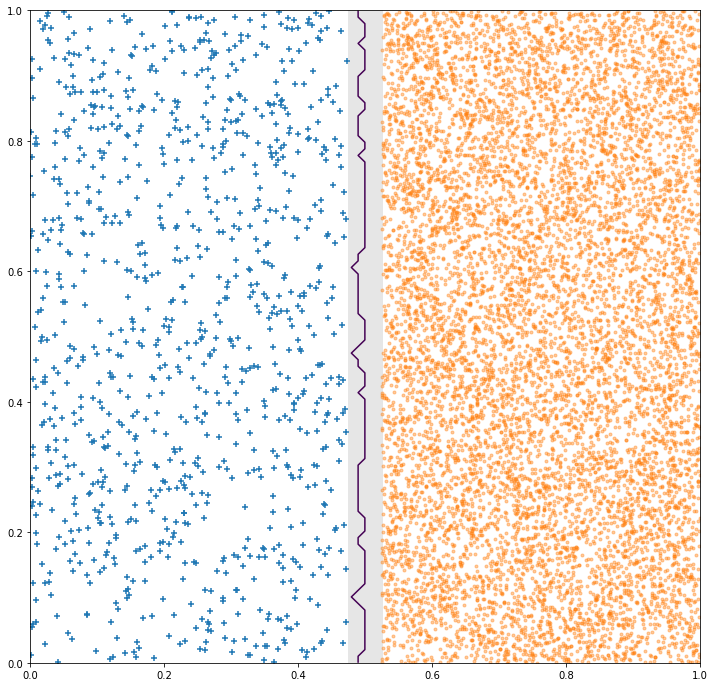@: centerimage ## 1-NN at a boundary (100$+$/ 1000$-$)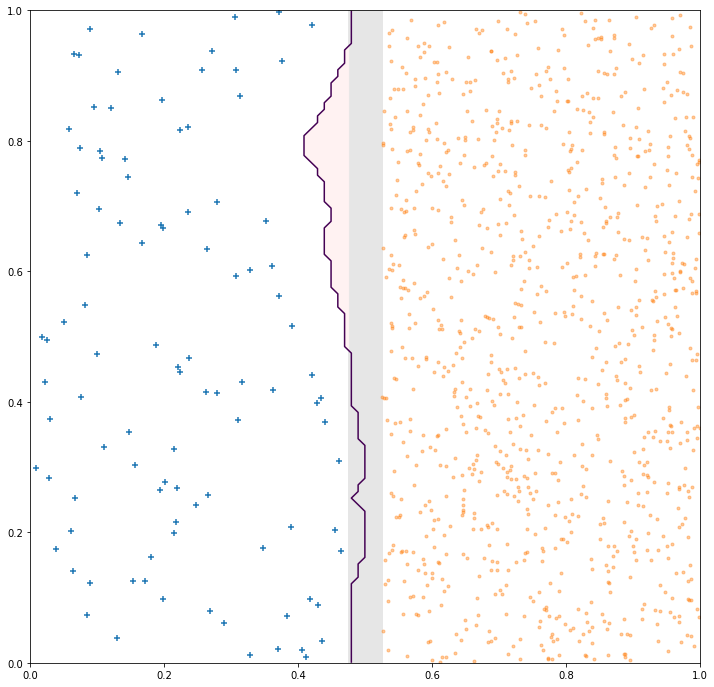@: centerimage ## 1-NN at a boundary (10$+$/ 100$-$)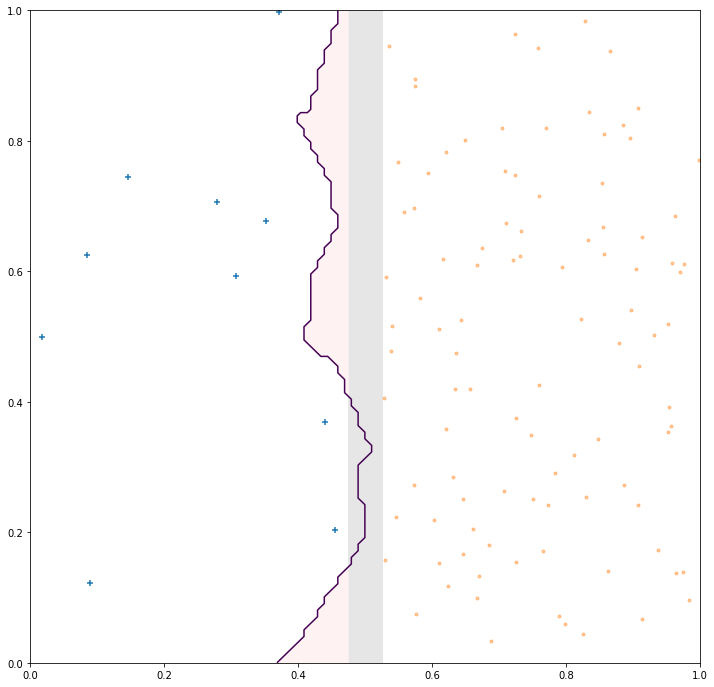## 11-NN: increasing k? // (from 1 to 11)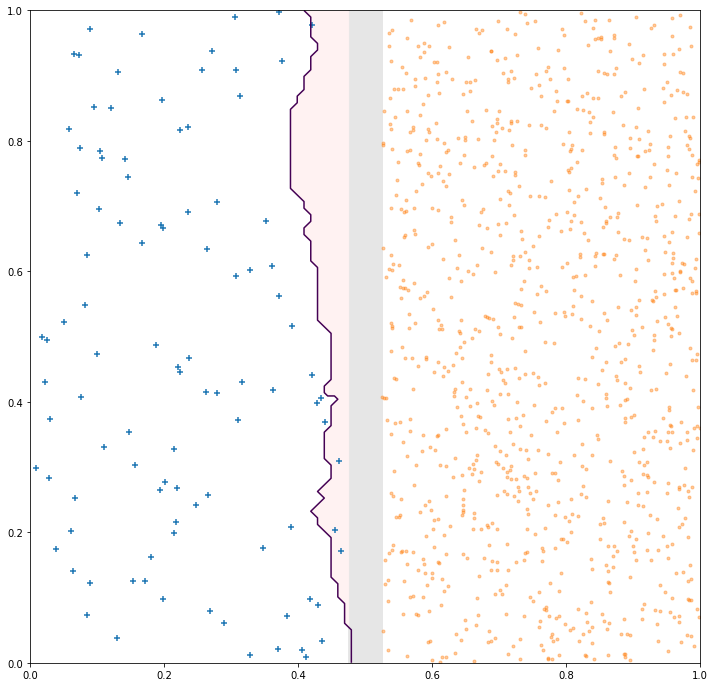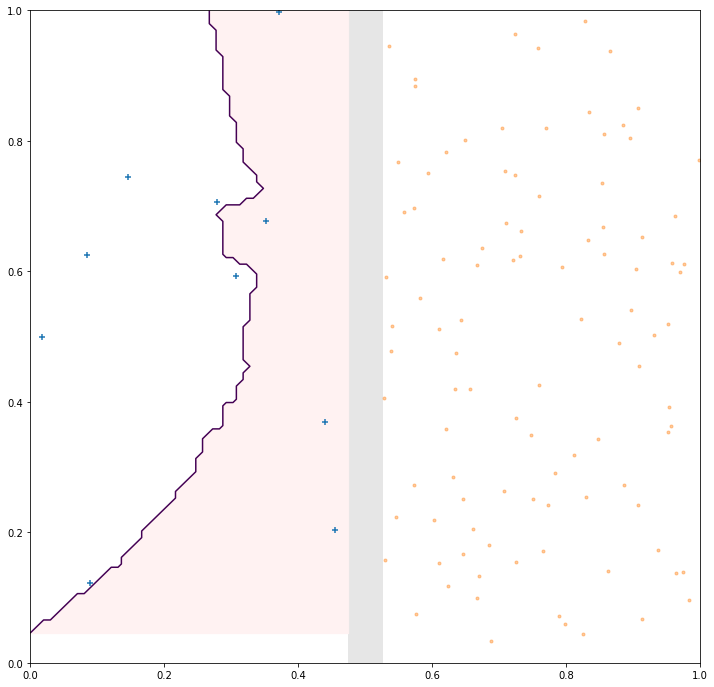- @anim: #NOTHING | div # @copy: gammann ##$\gamma$-NN Idea: push the decision boundary- Goal: correct for problems due to sampling with imbalance - Genesis: GAN to generate "$+$" around existing ones - ⇒ unstable, failing, complex @:no - Approach - artificially make$+$closer to new points - how? by using a different distance for$+$and$-$- the base distance to$+$gets multiplied by a parameter$\gamma$(intuitively$\gamma \le 1$if$+$is rare) \def{\x}{\mathbb{x}} d_\gamma(\x,\x_i) = \begin{cases} d(\x,\x_i) & \text{if} \; \x_i\in S_-,\\ \gamma \cdot d(\x,\x_i) & \text{if} \;\x_i\in S_+. \end{cases} ##$\gamma$-NN: varying$\gamma$with two points@anim: #g78,#g146 | #g66,#g134 | #g56,#g122 | #g46,#g110 | #g88,#g160 ##$\gamma$-NN: varying$\gamma$with a few$+$-$\gamma$-NN can control how close to the minuses it pushes the boundary ##$\gamma$-NN: Algorithm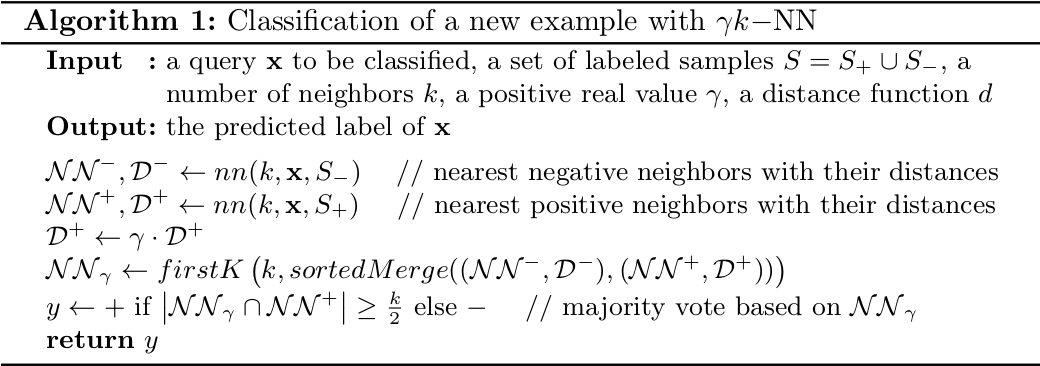- Trivial to implement - Same complexity as k-NN (at most twice) - Training - none, as k-NN -$\gamma$is selected by cross-validation (on the measure of interest) ##$\gamma$-NN: a way to reweight distributions - In uncertain regions // when we have more and more points - At the boundaries // depends on intrisic dimensionality @:/no-status ## Results on public datasets (F-measure)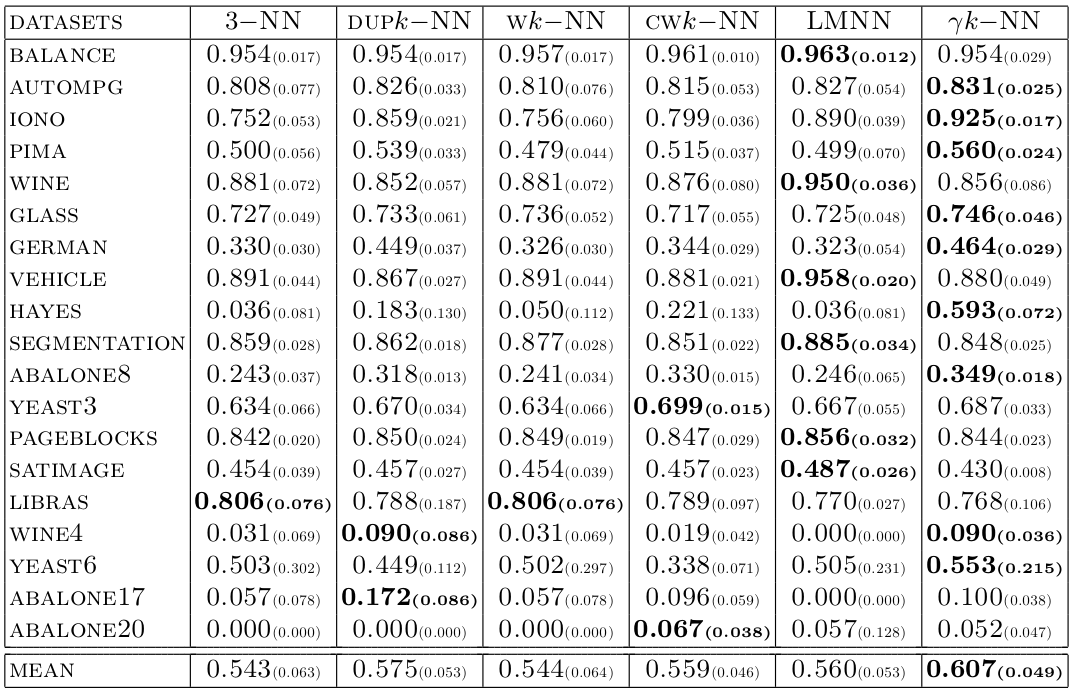@:/no-status ## Results on DGFiP datasets (F-measure) // underline = second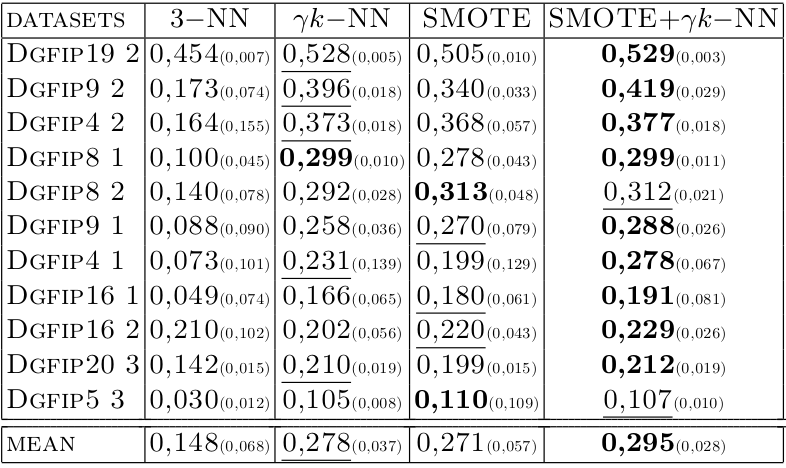##$\gamma$-NN at a boundary (10 and 100$+$)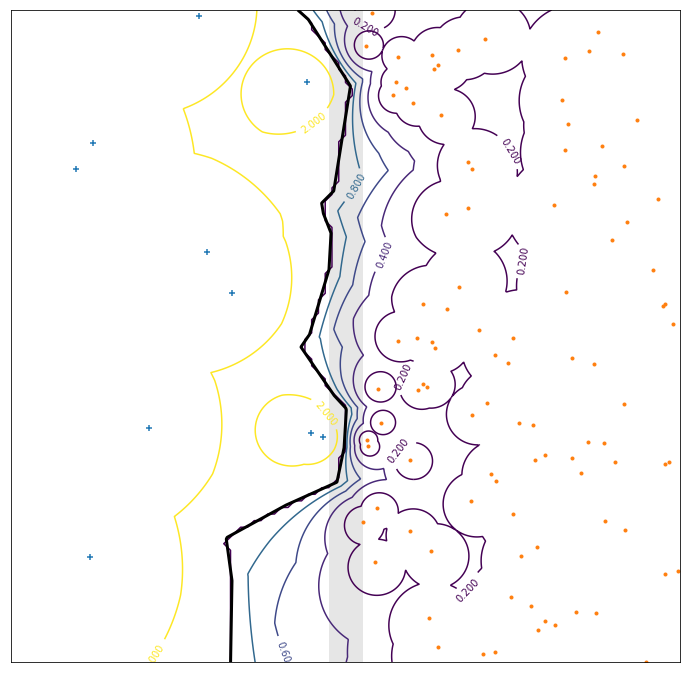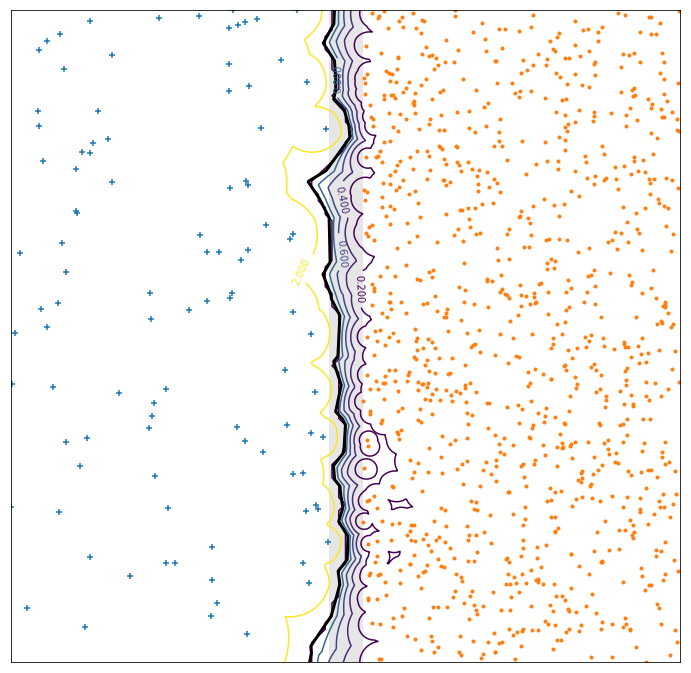## (some) Work in progress - Note: -$\gamma$-NN learns a metric for comparing a query to a$+$-$\gamma$-NN kind of learn the size of a sphere around$+$- this is “Metric Learning” - Extension - learn a full metric (a matrix$M$and not only$\gamma$) - derive a learning algorithm (not just cross-validation) ##@eval-header: return highlightLi($o.s, 5) # @copy: overview @:paperattrib no-libyli ## From Cost-Sensitive Classification to Tight F-measure Bounds - **Kevin Bascol**, Rémi Emonet, Elisa Fromont, Amaury Habrard,
**Guillaume Metzler**, Marc Sebban    - AISTATS2019 ## Optimizing the $F_\beta$-measure? - Reminder {.padli .densemath} - Precision: $prec=\frac{TP}{TP+FP}$ - Recall: $rec=\frac{TP}{P} = \frac{TP}{TP+FN}$ - $F_\beta$-measure: $F_\beta = (1+\beta^2)\frac{prec\cdot rec}{\beta^2 \cdot prec + rec}$ - **Non-separability**, i.e.    $F_\beta \ne \sum_{(x_i, y_i) \in S}...$
*NB: accuracy is separable, $acc = \sum_{(x_i, y_i) \in S} \frac{1}{m} \delta(y_i - \hat{y_i})$ @:denser* - ⇒ The loss for one point depends on the others {.no} - ⇒ Impossible to optimize directly {.no} - ⇒ Impossible to optimize on a subset (minibatch) {.no} ## Weighted classification for $F_\beta$ - $F_\beta = \frac{(1+\beta^2)\cdot (P - FN)}{1 + \beta^2 P - FN + FP} = \frac{(1+\beta^2)\cdot (P - e_1)}{1 + \beta^2 P - e_1 + e_2}$

{.no} - The $F_\beta$-measure is linear fractional *(in $e = (e_1, e_2) = (FN, FP)$) @:dense* - i.e. $F_\beta = \frac{\langle a', e\rangle + b}{\langle c, e\rangle + d} = \frac{A}{B}$ {.no} - Relation to weighted classification - $\hphantom{\Leftrightarrow } F_\beta \ge t$      (we achieve a good, above $t$, $F_\beta$ value) {.no} - $\Leftrightarrow A \ge t\cdot B$ {.no} - $\Leftrightarrow A - t\cdot B \ge 0$ {.no} - $\Leftrightarrow (1+\beta^2)\cdot (P - e_1) - t ( 1 + \beta^2 P - e_1 + e_2) \ge 0$ {.no} - $\Leftrightarrow (- 1 - \beta^2 + t) e_1 - t e_2 \ge - P (1 + \beta^2) + t ( 1 + \beta^2 P)$ {.no} - $\Leftrightarrow (1 + \beta^2 - t) e_1 + t e_2 \le - P (1 + \beta^2) + t ( 1 + \beta^2 P)$ {.no} - ⇒ so, we can minimize the weighted problem
with class weights $a(t) = (1 + \beta^2 - t, t)$ {.no} # CONE Demo... - contact me to know if the demo is publicly available yet @eval-header: $o.s++ @eval-header: return highlightLi($o.s) # @copy: overview # Learning normality ## Unsupervised Temporal Motif Mining
in videos / temporal data (spectrograms, ...)@anim: #layer1 + -#init | #layer2 | #layer3 | #layer6 | #layer7 | #layer4 | #layer5 ## Temporal Patterns in Videos: Full Process- @anim: #layer1 | #shortll | #tdocetc | #shorttm | #layer7 | #layer5 - @anim: #layer6 + -#layer3 - @anim: -#layer6 + #layer2 ## Video Motif Representation {blacktspan}- @anim: #motiftable | #rt0 | #rt1 | #rt2 + #rt3 + #rt4 + #rt5 | #giffy | #arrow + #magic # Example Motifs Obtained from a Static Camera @:.img3inwidth ##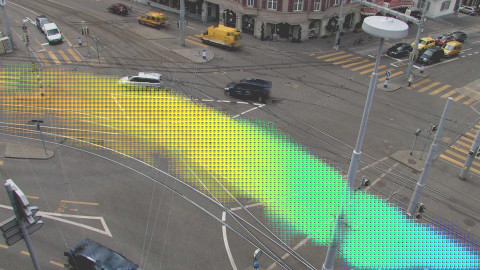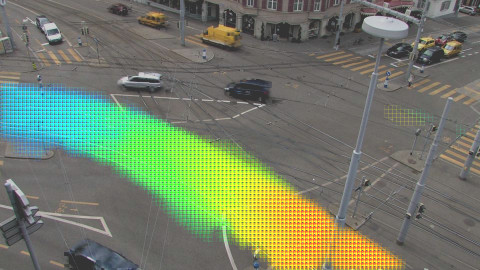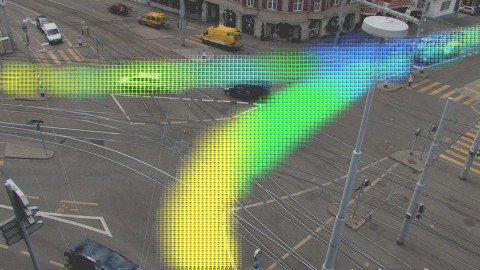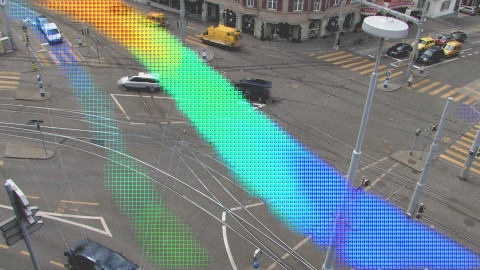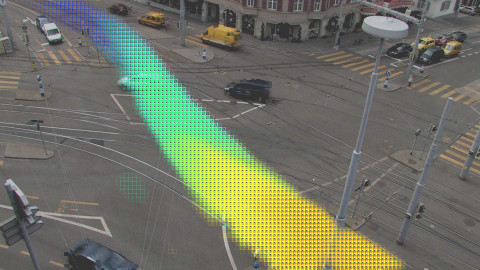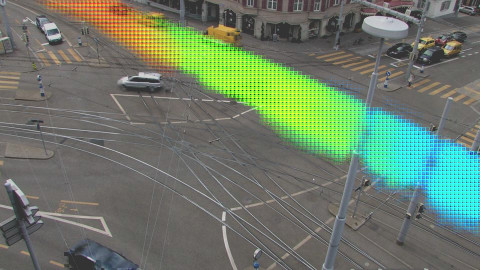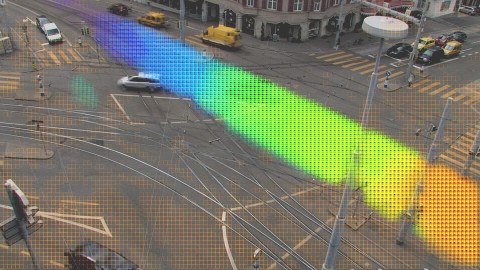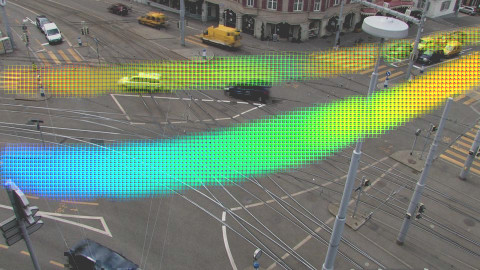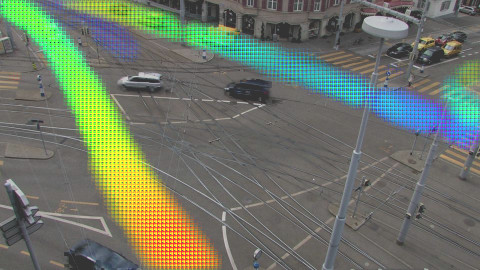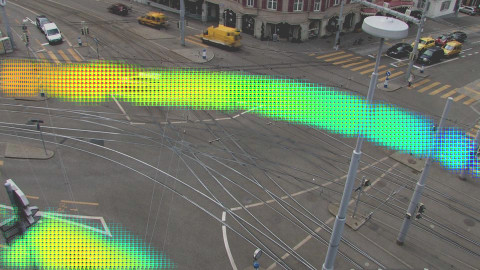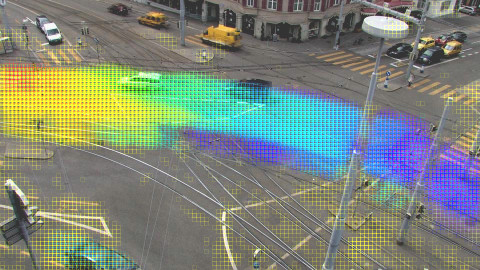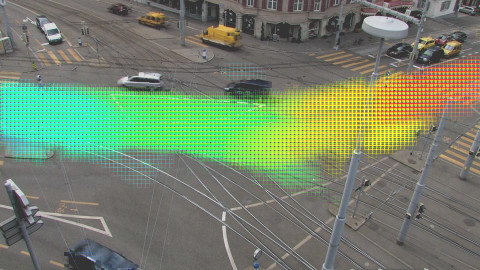@:.imagets ## Application with Static Cameras -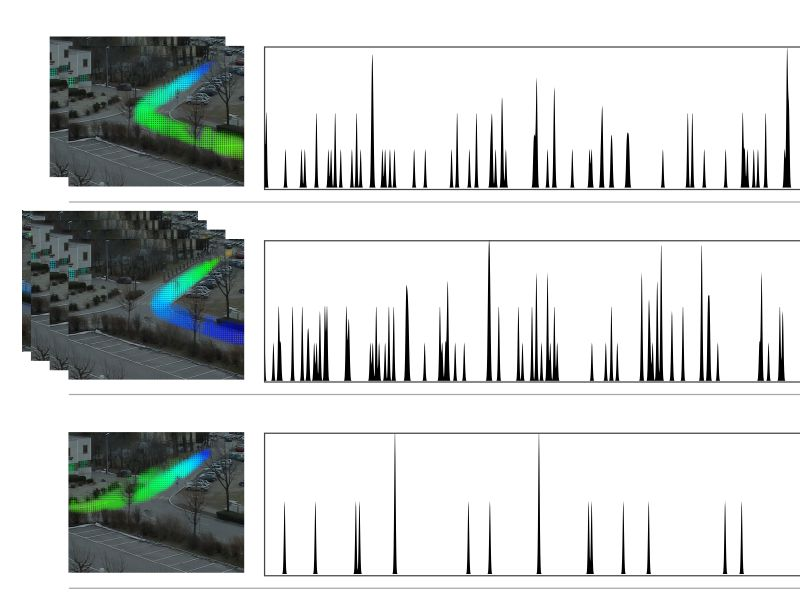scene understanding -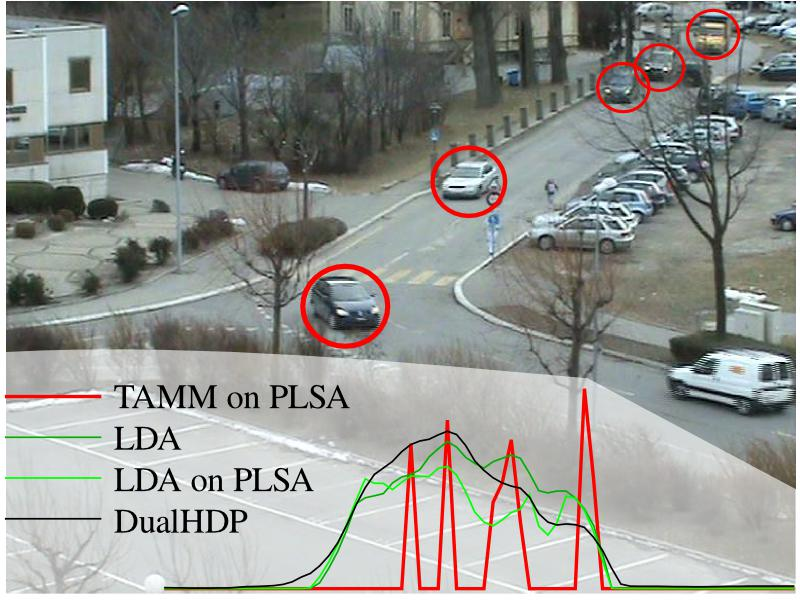car counting -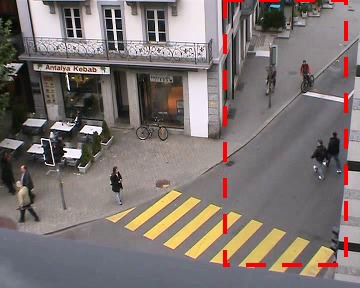anomaly detection -stream selection, anomaly detection,  multi-camera analysis @:.imagets ## Audio data? -a pair of microphones… -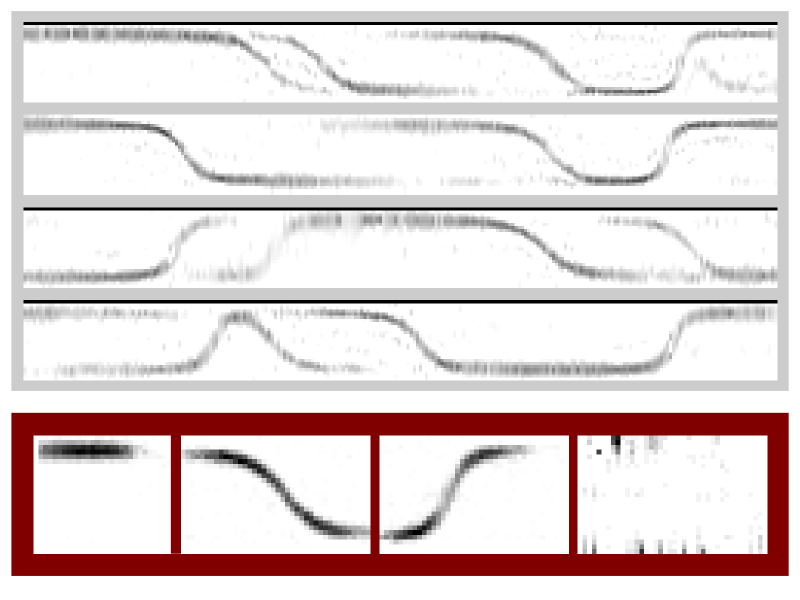… meaningful motifs… -… and good counting results - ... also with spectrograms # How Can We Do This?! ## Sol. 1: Hierarchical Probabilistic Models $\hspace{1cm}\mathcal{L} = \sum\_d \sum\_w \sum\_{t\_a} n(w,t\_a,d) log \sum\_z \sum\_{t\_s} p(w,t\_r|z) p(z,t\_s|d)$ {center}- Generative Model
⇒ interpretable by design - ⚠ unknown number of motifs
⇒ use infinite models - Inference - maximum likelihood, EM like - Sparsity on occurrences $p(ts|z, d)$ - new objective function: \ $\mathcal{L} - \lambda\_{sparse} \sum\_d \sum\_z KL(U || p(ts|z, d))$ ## Sol. 2: Neural Networks, Auto-encoders - Principle of auto-encoders - learn to produce the input from the input - going through a limited-size representation (bottleneck) - $x' = f(x) = f_{DEC}(f_{ENC}(x))$ - minimize the reconstruction error $d(x, x') = \left\\|x - x'\right\\|^2$ - ⚠ Issues: interpretability, number of motifs, ...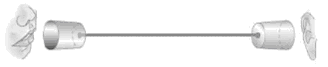## Sol. 2: Interpretable Auto-Encoders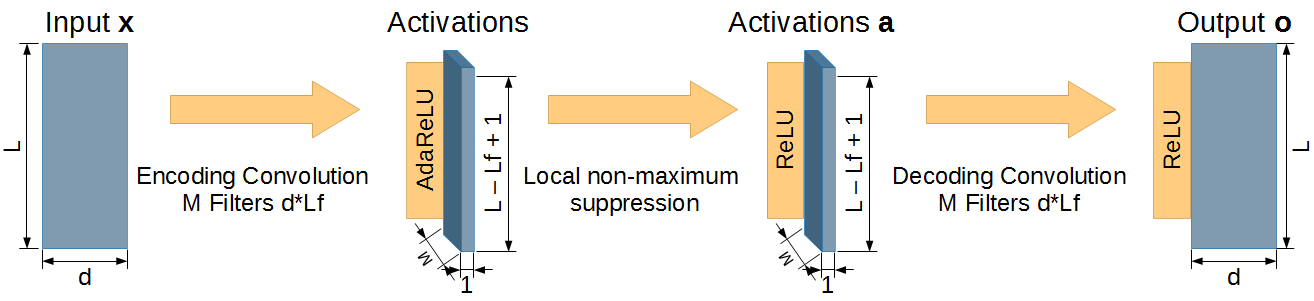- Add specific operators (layers) - global specialized maximum selection (AdaReLU) - locally, filter response decorrelation - Special Loss: a combination of well-chosen target functions - encourage sparse motifs (with a lot of zero) - encourage sparse activations - ⚠ unknown number of motifs ⇒ use “group-sparsity” @eval-header: $o.s++ @eval-header: return highlightLi($o.s) # @copy: overview @:/title-slide # Thank you! Questions?{.captain}@sticky-add: @off # MORE (from before but there is a lot more more in 2019-10-17) - idea: new robust distance: min l_inf dist on a subset of features,, or min l2 on a fixed-size subset of features ( subset fixed or not ?)